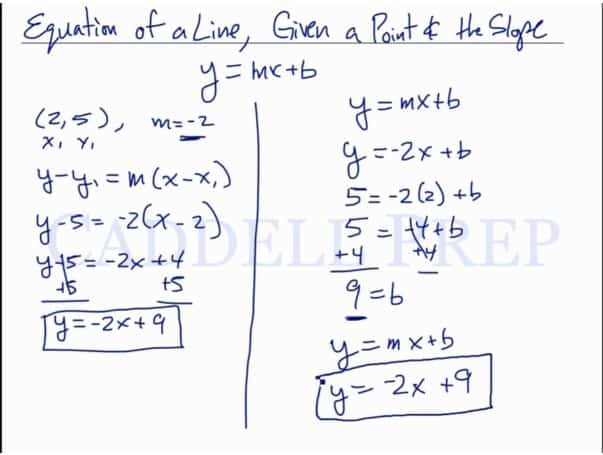This video explains how to find the equation of a line, given a point and the slope of the line. After you finish this lesson, view all of our Algebra 1 lessons and practice problems.

There are two ways of solving this.

One way is using the slope-intercept formula, y = mx + b

And the other way is by using the point-slope formula,$y - y_1 = m(x - x_1)$

## Example of Finding The Equation Of A Line, Given The Slope And A Point

### Example 1

Find the equation of the line that passes through the point$( -2 , 6 )$ with a slope of$3$.

Let’s solve this using the point-slope formula which is$y - y_1 = m (x - x_1)$

Now, let’s substitute$y - (6) = 3 (x - (-2))$$y - (6) = 3 (x +2)$

Now, let’s manipulate this to get the slope-intercept form$y - 6 = 3x + 6$

Now, let’s isolate$y$ by adding$6$ on both sides.$y - 6 +6 = 3x + 6+3$$y = 3x + 12$

### Example of 2

Find the equation of the line that passes through the point$( -5 , 2 )$ with a slope of$\dfrac{-3}{4}$.

Let’s solve this is using the slope intercept form directly.$y = mx + b$$y = \dfrac{-3}{4}x + b$

Multipy$4$ on both sides$4y = -3x + 4$

Let’s solve for$b$ by substituting the values of$x$ and$y$.$4(2) = \dfrac{-3}{-5} + 4b$$8 = 15 + 4b$

Then isolate$b$ by subtracting$15$ on both sides$8-15= 15 + 4b-15$$-7 = 4b$

Divide$4$ to isolate$b$$\dfrac{-7}{4} = \dfrac{4b}{4}$$\dfrac{-7}{4} = b$

Now let’s write our slope intercept form$y = \dfrac{-3}{4}x - \dfrac{7}{4}$

## Video-Lesson Transcript

Let’s go over finding the equation of a line, given a point and the slope.

We’re going to use the slope intercept formula:$y = mx + b$

For example:$(2, 5)$,$m = -2$

We’re going to solve this in two different ways.

First is the point-slope formula which is$y - y_1 = m (x - x_1)$

Now, let’s substitute$y - 5 = -2 (x - 2)$

Now, let’s manipulate this to get the slope intercept form$y - 5 = -2x + 4$

Now, let’s isolate$y$ by adding$5$ on both sides.$y - 5 + 5 = -2x + 4 + 5$$y = -2x + 9$

Or the second way to solve this is to do the slope intercept form directly.$y = mx + b$$y = -2x + b$

Let’s solve for$b$ by substituting the values of$x$ and$y$.$5 = -2(2) + b$$5 = -4 + b$

Then isolate$b$ by adding$4$ on both sides$5 + 4 = -4 + 4 + b$$9 = b$

Now let’s write our slope intercept form$y = -2x + 9$

which is the same as our first answer.